Type
Solution Manual
Book Title
Principles of Operations Management: Sustainability and Supply Chain Management 10th Edition
ISBN 13
978-0134181981

### 978-0134181981 Module B Part 1

February 27, 2020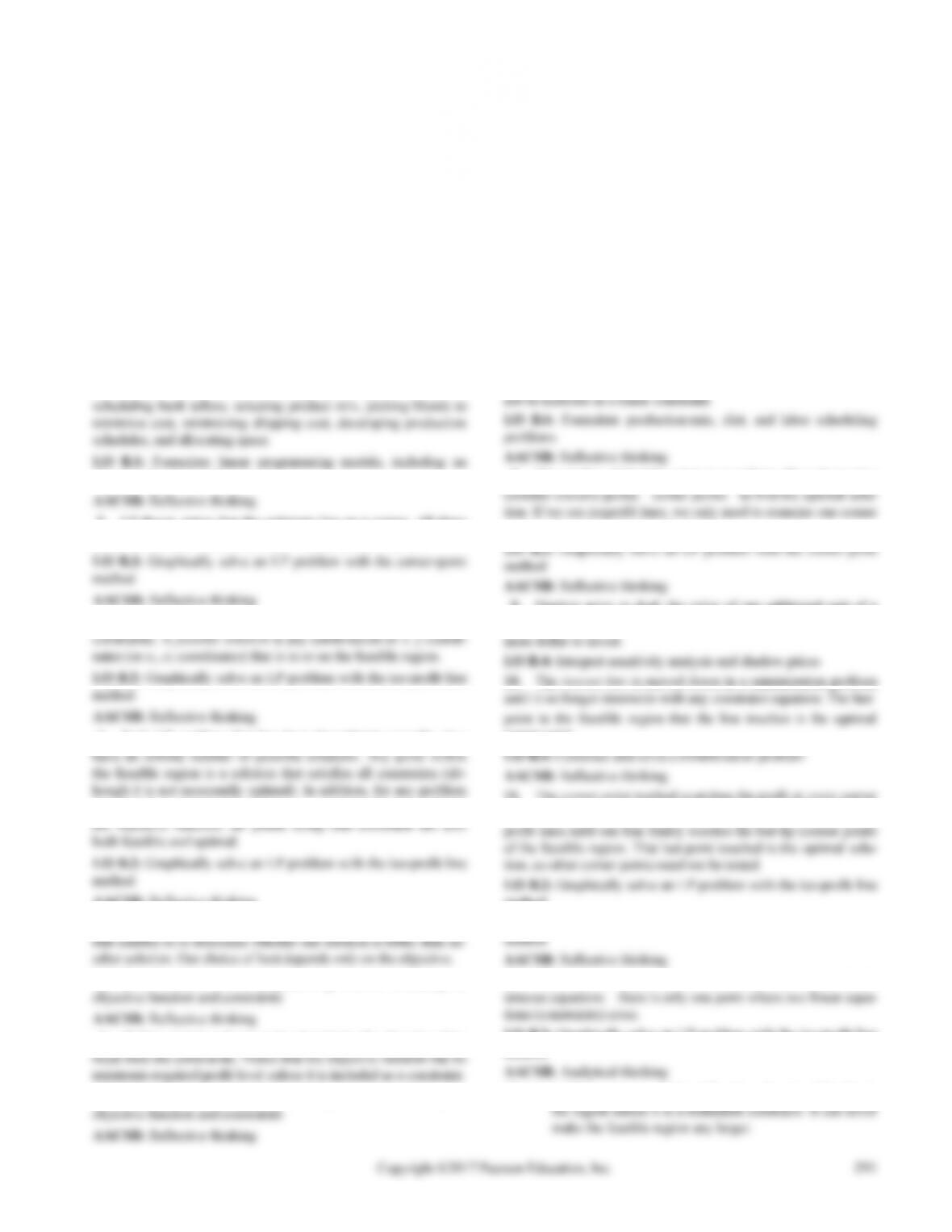B
B U S I N E S S A N A L Y T I C S M O D U L E
Linear Programming
DISCUSSION QUESTIONS
1. Students may select from eight LP applications given in the
introduction: school bus scheduling, police patrol allocation,
objective function and constraints
2. LP theory states that the optimum lies on a corner. All three
solution techniques make use of the “corner point” feature.
3. The feasible region is the area bounded by the set of problem
4. Each LP problem that has been formulated correctly does
in which the optimal solution lies on a constraint that is parallel to
AACSB: Reflective thinking
5. The objective function contains the profit or cost information
LO B.1: Formulate linear programming models, including an
6. Before activity values can be placed into the objective, they
LO B.1: Formulate linear programming models, including an
7. As long as the costs do not change, the diet problem always
provides the same answer. In other words, the diet is the same
every day. Unlike animals, people enjoy variety, and variety can-
8. The number of feasible solutions is infinite. We only need to
point to determine the optimal solution.
9. Shadow price or dual: the value of one additional unit of a
resource, such as one more hour of a scarce labor resource or one
corner point.
point, whereas the iso-profit line method draws a series of parallel
method
LO B.3: Graphically solve an LP problem with the corner-point
12. When two constraints do not cross at an axis, we use simul-
LO B.2: Graphically solve an LP problem with the iso-profit line
13. (a) Adding a new constraint will reduce the size of the feasi-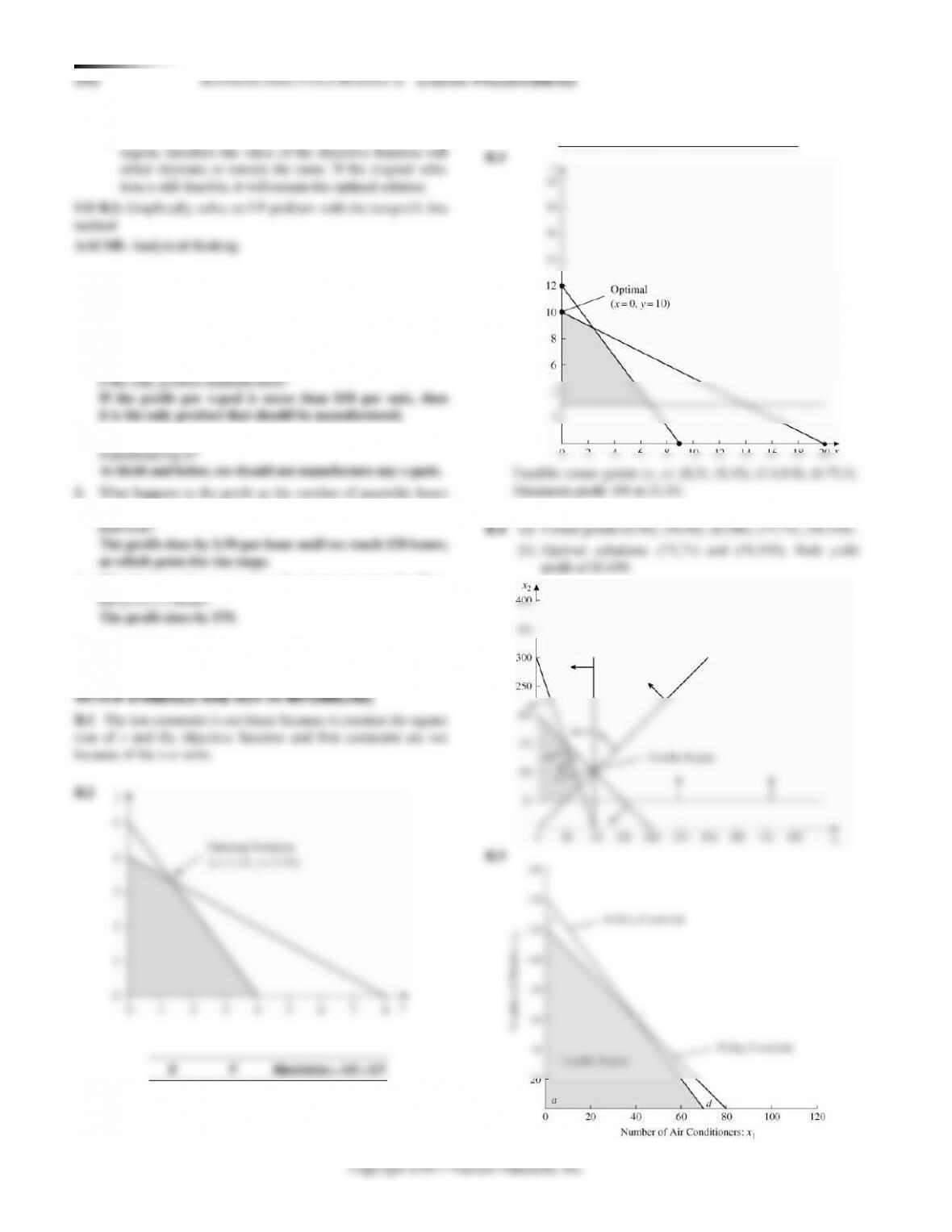(b) A new constraint can only reduce the size of the feasible
ACTIVE MODEL EXERCISE (AVAILABLE IN
MYOMLAB)
ACTIVE MODEL B.1: LP Graph
1. By how much does the profit on x-pods need to rise to make
2. By how much does the profit on x-pods need to fall to stop
increases by 1 hour at a time? For how many hours does this
4. What happens if we can reduce the electronics time for Blue-
END-OF-MODULE PROBLEMS (PROBLEMS WITH
ASTERISKS ARE IN MYOMLAB ONLY; PROBLEMS
0
0
0
4
0
16
0
4
24
1.33
3.33
25.33 (optimal)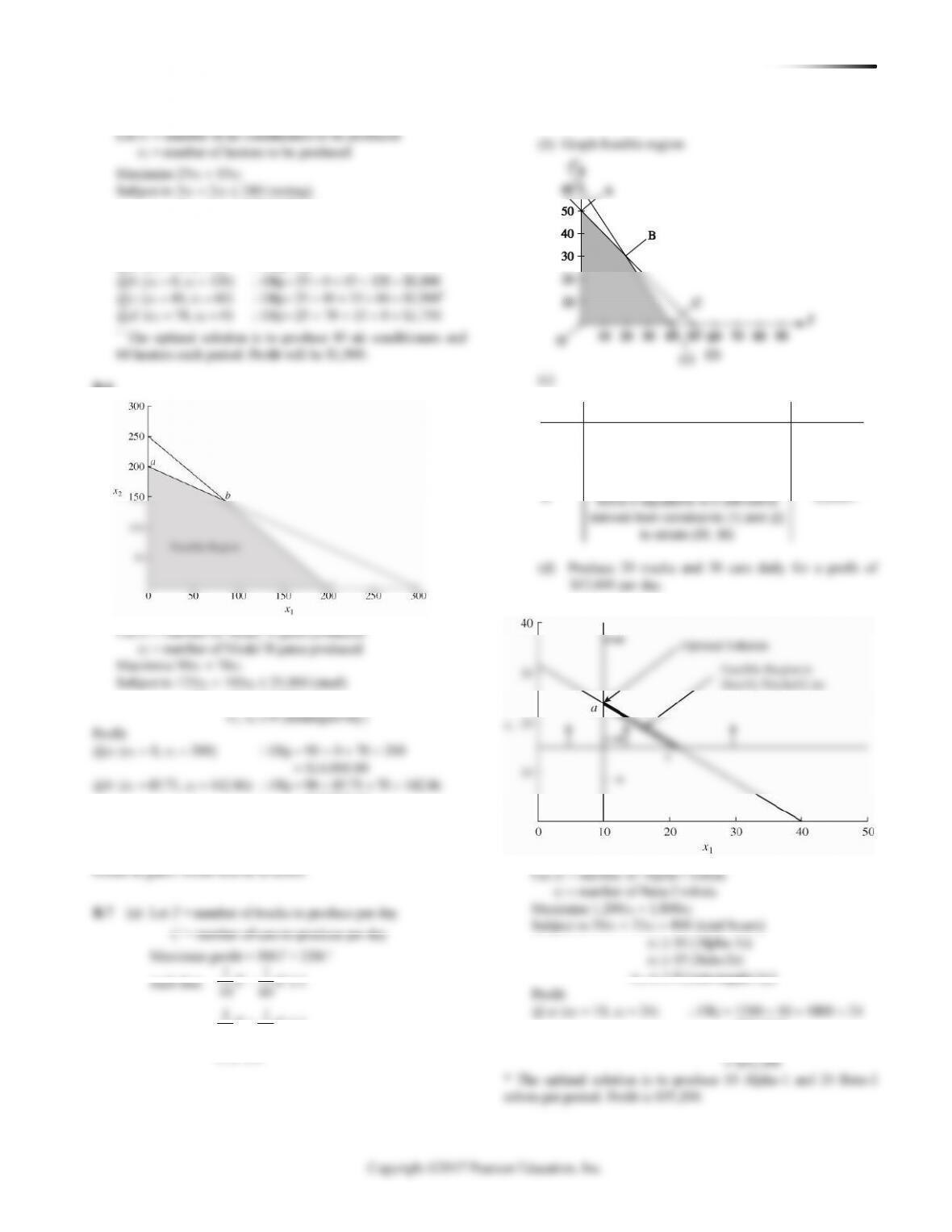BUSINESS ANALYTICS MODULE B LI N E A R PR O G R A M M I N G 293
2x1 + 1x2 140 (drilling)
x1, x2 0 (non-negativity)
Profit:
B.6
20x1 + 30x2 6,000 (zinc)
= \$17,714.10
@c: (x1 = 200, x2 = 0) Obj = 90 200 + 70 0
= \$18,000.00*
* The optimal solution is to produce 200 Model A gates, and 0
B.8
= \$55,200*
@ b: (x1 = 21.25, x2 = 15) Obj = 1200 21.25 + 1800 15
+1
50 50
TC
Point
Coordinates
Profit
O
(0, 0)
0
A
(0, 50)
11,000
C
(40 ,0)
12,000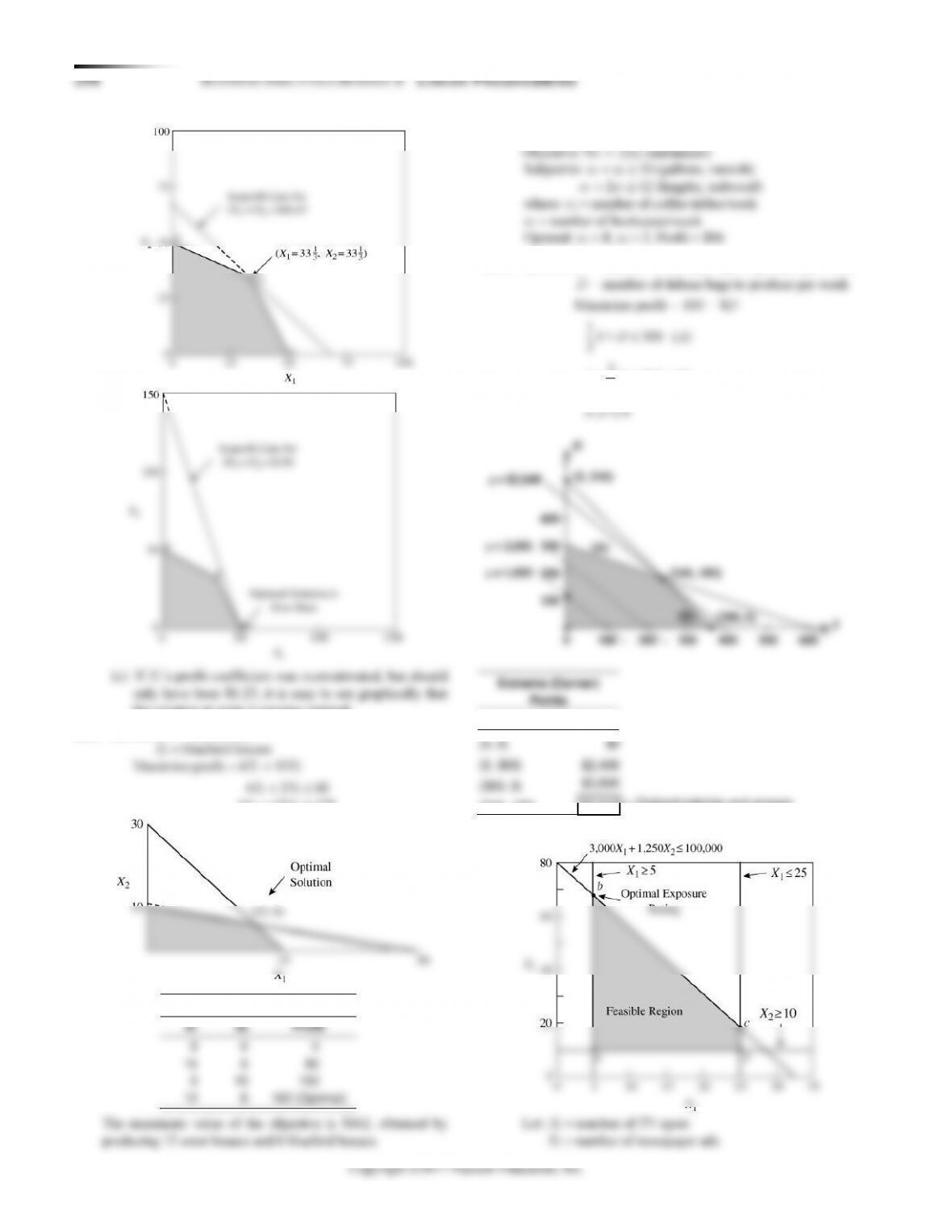the solution at point b remains optimal.
B.10 (a) Let X1 = wren houses
4X1 + 12X2 120
Corner Points
B.11 The original equations are:
B.12 (a, b) Let S = number of standard bags to produce per week
Point
Profit
(240, 180)
\$3,840
(b)
B.9 (a)
(b)
3
S D B
+
B.13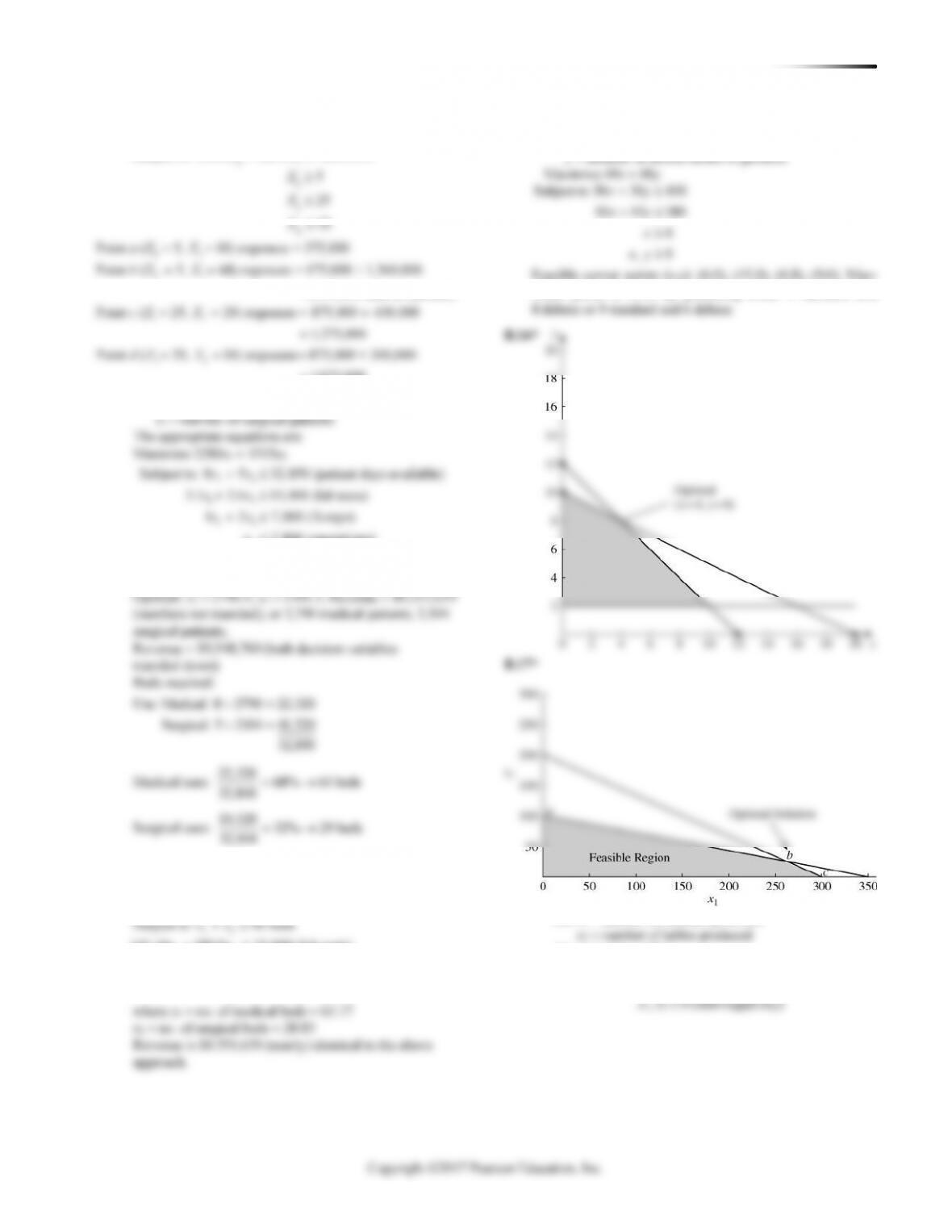BUSINESS ANALYTICS MODULE B LI N E A R PR O G R A M M I N G 295
Maximize exposures = 35,000X1 + 20,000X2
=
1,075,000
B.14 Let x1 = number of medical patients
2
1
2,800 (operations)
x
x
2
, 0 (non-negativity)x
Here is an alternative approach that solves directly for the
number of beds:
Maximize revenues = 104,025x1 + 110,595x2
+
+
12
12
2
141.44 189.8 15,000 (lab tests)
45.63 146 7,000 ( -rays)
X
73 2,800 (operations)
xx
xx
x
B.15* Let x = number of standard model to produce
Maximize 9x1 + 20x2
Subject to 4x1 + 6x2 1,200 (hours)
10x1 + 35x2 3,500 (board-feet)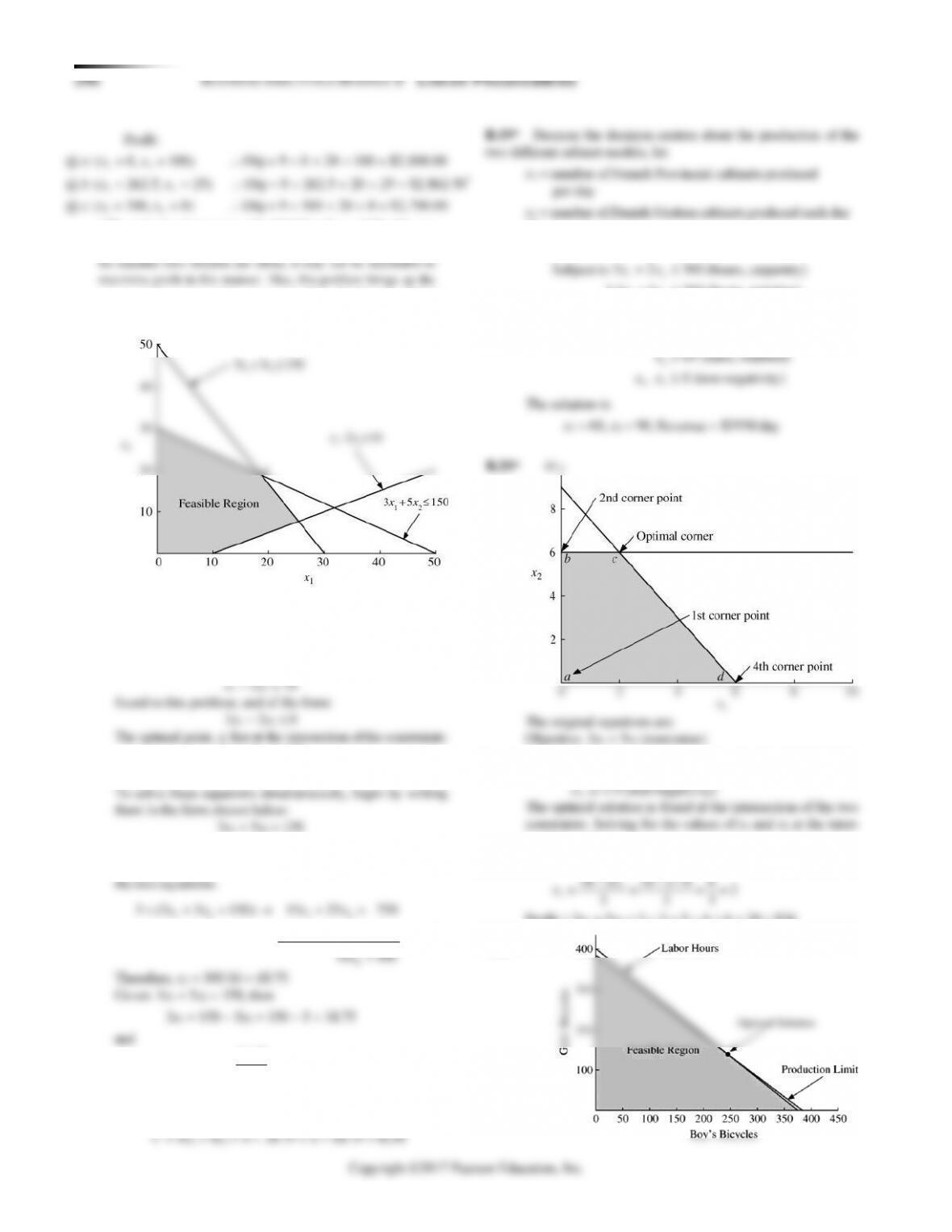Note that this problem has one constraint with a negative
sign. This may cause the beginning student some confu-
sion in plotting the constraint.
This would probably be a good time to stress the plot-
ting of equations of the form:
3x1 + 5x2 150
5x1 + 3x2 150
5x1 + 3x2 = 150
Multiply the first equation by 5, the second by 3, and add
+ = =
1 2 1 2
3 (5 3 150) 15 9 450
x x x x
1
56.25 18.75
3
x==
Thus, the optimal solution is: x1 = 18.75, x2 = 18.75
The profit is given by:
The equations become:
Objective: 28x1 + 25x2 (maximize revenue)
12
12
1
1.5 1 200 (hours, painting)
0.75 0.75 125 (hours, finishing)
60 (units, contract)
xx
xx
x
+
+
Subject to x2 6
3x1 + 2x2 18
section, we have:
x2 = 6
Profit = 3x1 + 5x2 = 3 2 + 5 6 = 6 + 30 = \$36
* The optimal solution is to make 262.5 benches and 25 tables per
period. Profit will be \$2,862.50. Because benches and tables may
proper interpretation of the statement “One should make 262.5
(a fractional quantity) benches per period.”
B.18*
B.21*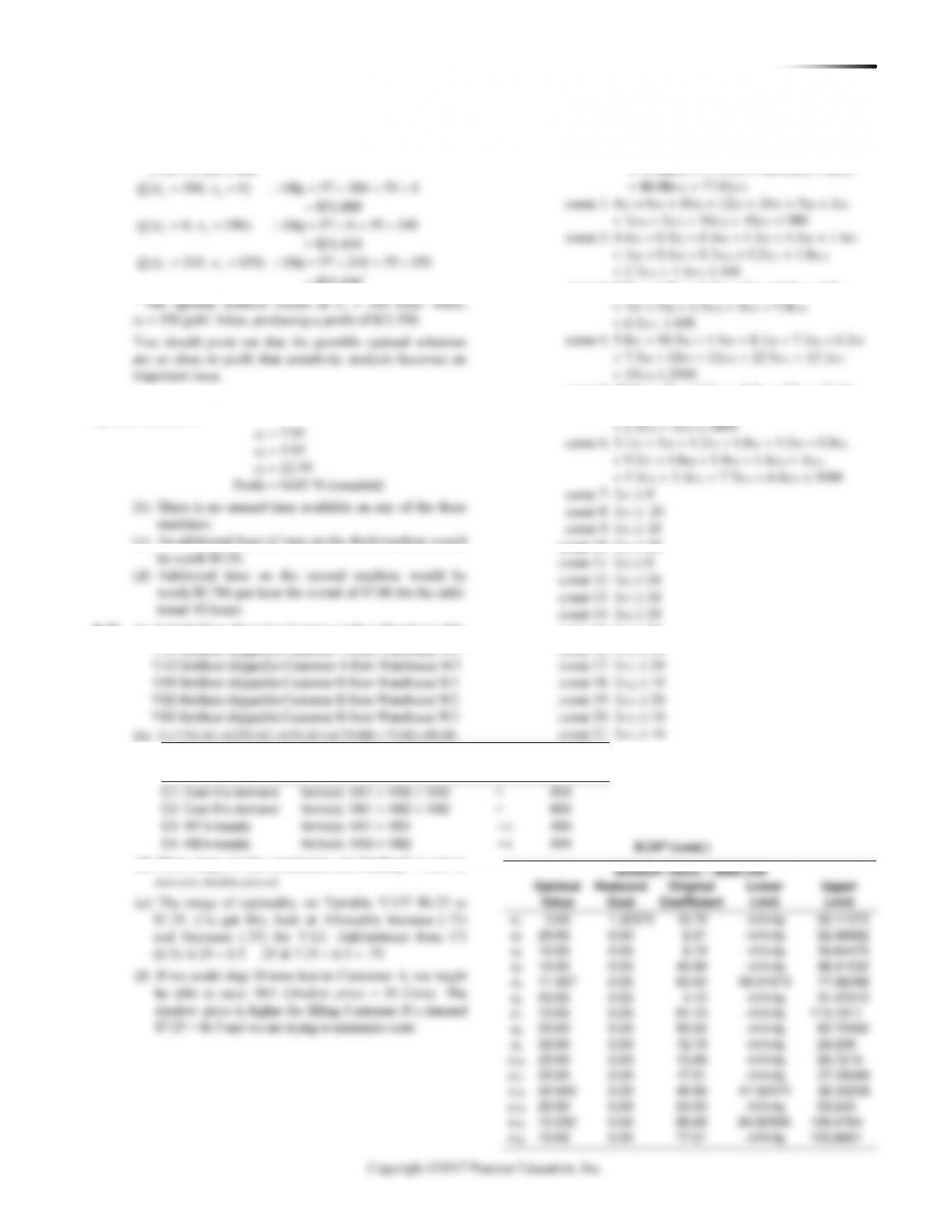BUSINESS ANALYTICS MODULE B LI N E A R PR O G R A M M I N G 297
Maximize: 57x1 + 55x2
Subject to: x1 + x2 390
B.22 (a) Using POM for Windows software, we find that the
optimal solution is:
B.23 (a) VA1 fertilizer shipped to Customer A from Warehouse W1
B.24* (a) Maximize 18.79x1 + 6.31x2 + 8.19x3 + 45.88x4 + 63x5
+ 4.1x6 + 81.15x7 + 50.06x8 + 12.79x9
const 3: 0.7x1 + 1.8x2 + 1.5x3 + 2x4 + 1.2x5 + 1.5x6
const 5: 10.9x1 + 2x2 + 2.3x3 + 4.9x5 + 10x6 + 11.1x7
+ 12.4x8 + 5.2x9 + 6.1x10 + 7.7x11 + 5x12
const 15: 1x9 50
(c)
Description
Variables and Coeffi-
cients?
What Type?
RHS?
C5: W3’s supply
+
=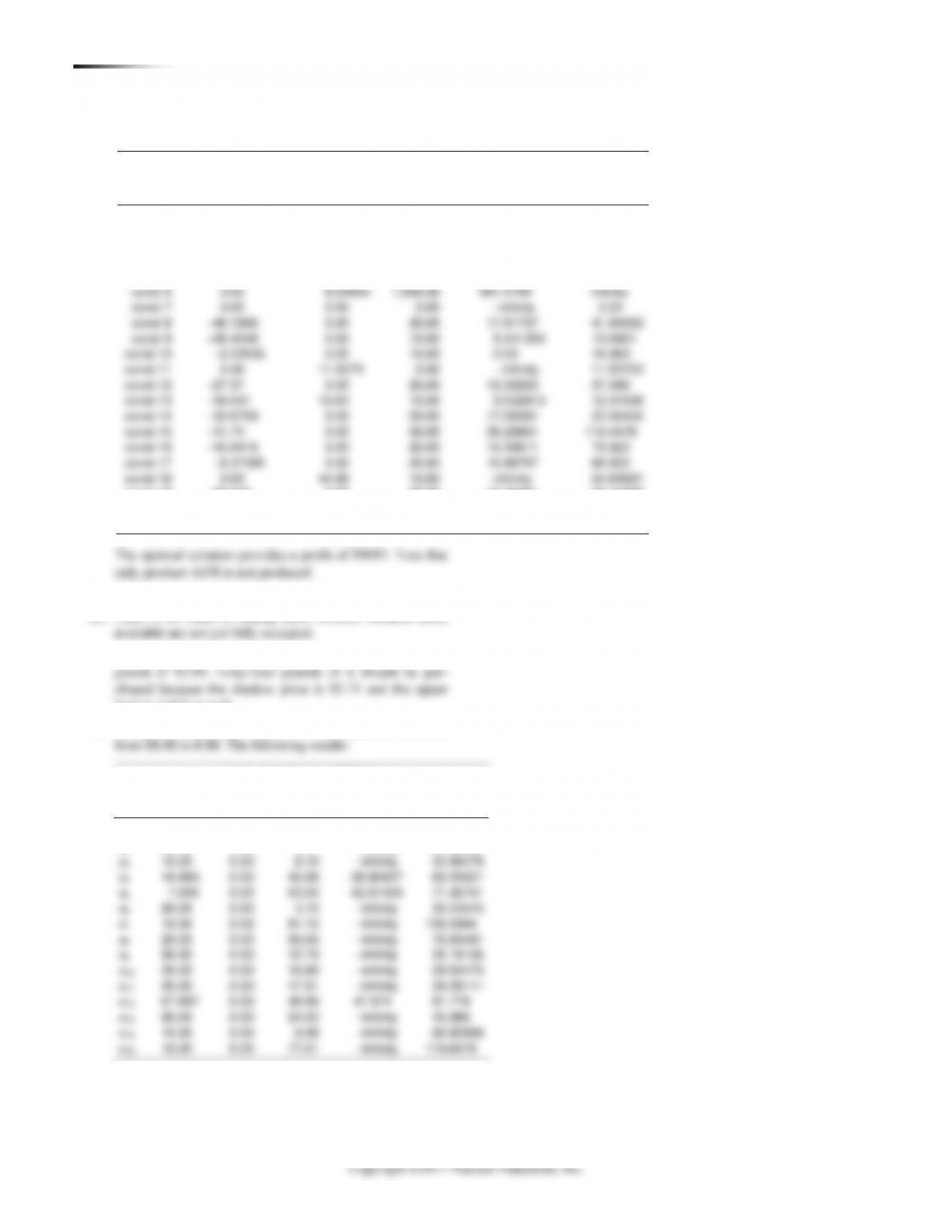298 BUSINESS ANALYTICS MODULE B LI N E A R PR O G R A M M I N G
Solution Value = 9683.229
Slack or
Original
Lower
Upper
Prices
Surplus
RHS
Limit
Limit
const 1
2.711812
0.00
980.00
861.5504
1,024.236
const 2
0.00
113.866
400.00
286.1337
Infinity
const 3
10.6486
0.00
600.00
587.7851
608.5712
const 4
2.182708
0.00
2,500.00
1,889.72
2,534.683
const 5
0.00
258.885
1,800.00
1,541.115
Infinity
const 19
29.243
0.00
20.00
15.45261
22.44298
const 20
0.00
2.20215
10.00
Infinity
12.20215
const 21
48.87
0.00
10.00
8.355577
12.84913
(b) The shadow prices are given in the table above.
(d) Two tons of steel at a total cost of \$8,000 implies a cost per
limit is 1,024 pounds.
(e) Change coefficient for variable x14 in objective function
Solution Value = 8865.5
Optimal
Reduced
Original
Lower
Upper
Value
Cost
Coefficient
Limit
Limit
x1
0.00
1.23911
18.79
Infinity
20.02911
B.24* (cont.)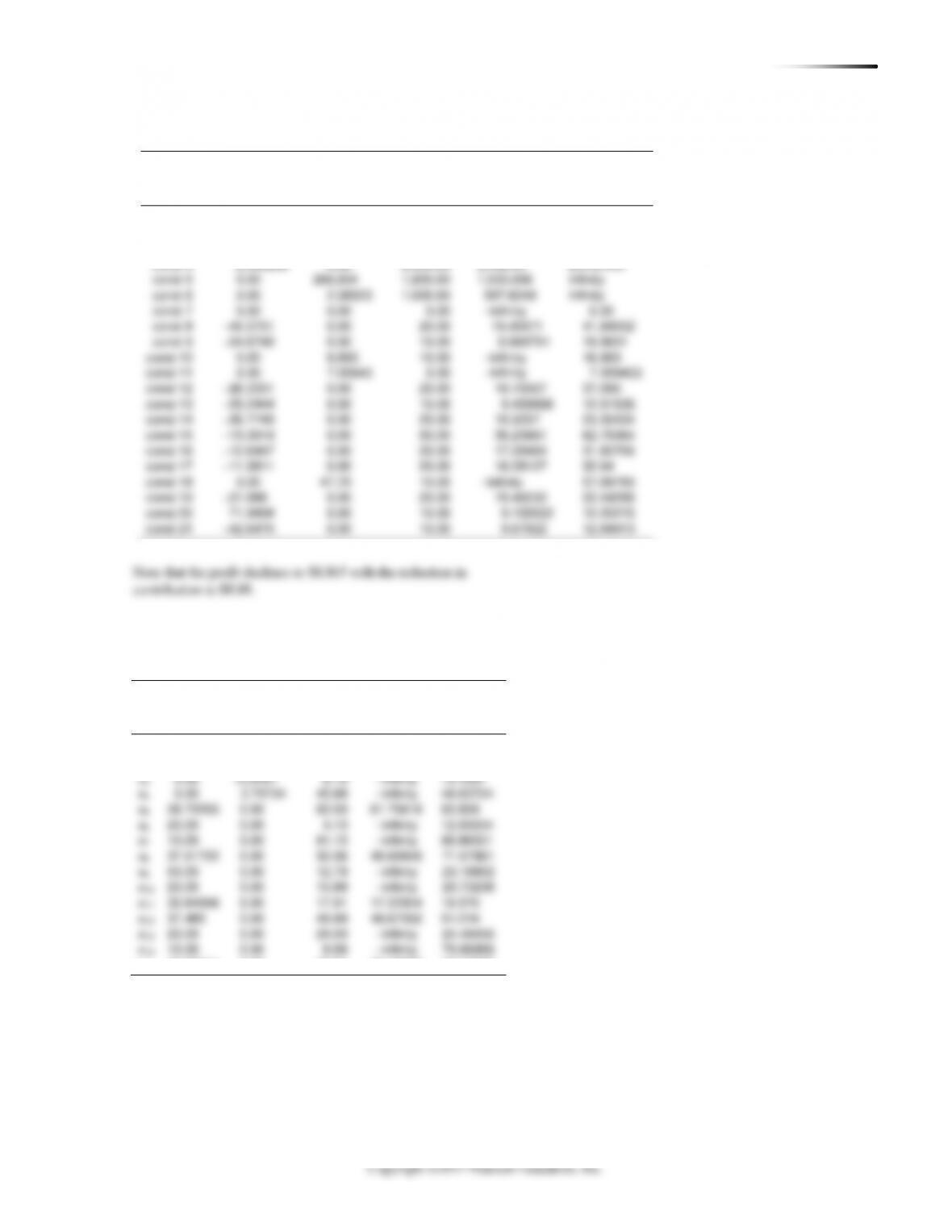BUSINESS ANALYTICS MODULE B LI N E A R PR O G R A M M I N G 299
Solution Value = 8865.5
Slack or
Original
Lower
Upper
Prices
Surplus
RHS
Limit
Limit
const 1
2.74856
0.00
980.00
913.6641
993.1374
const 2
0.00
113.879
400.00
286.1211
Infinity
const 3
9.197201
0.00
600.00
587.7851
601.577
B.24* (cont.)
(f) Constraints 7 through 11 become: x1 0, x2 0, x3 0,
x4 0, x5 0. The following results:
Solution Value = 9380.23
Optimal
Reduced
Original
Lower
Upper
Value
Cost
Coefficient
Limit
Limit
x1
0.00
7.90441
18.79
Infinity
26.69441
x2
0.00
16.81
6.31
Infinity
23.1219
x15
10.27741
0.00
77.01
75.18908
77.47366